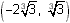Lesson 1

13.1.1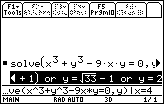The points on the graph of x3 + y3 – 9xy = 0 when x = 4 are (4, 2),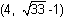, and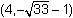.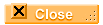Lesson 2

13.2.1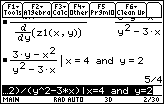The slope of the tangent line to the folium at (4, 2) is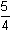13.2.2 The figure below shows that dy/dx = 0 at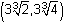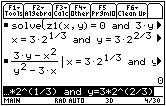13.2.3 The points of the folium that satisfy the system are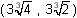and (0, 0).13.2.4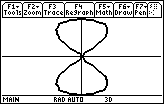13.2.5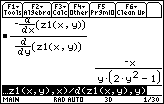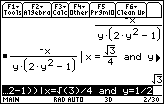The slope of y4y2 + x2 = 0 at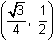is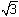.13.2.6 The points on y4y2 + x2 = 0 where the tangent is vertical are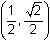,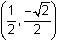,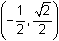,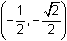.Self Test

The relation is shown in the following Viewing Cube:

 eye= –90 xmin = –6 ymin = –6 zmin = –10 eye= 0 xmax = 6 ymax = 6 zmax = 10 eye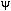= 0 xgrid = 14 ygrid = 14 ncontour = 5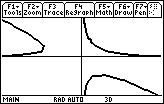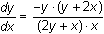The slope of the tangent at the point (1, 2) is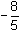The point where the tangent line is horizontal is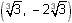The point where the tangent line is vertical is